# Fractions

Last Updated on October 4, 2018 by Alabi M. S.

MATHEMATICS

FIRST TERM

FIFTH WEEK

BASIC 6

THEME:

TOPIC: Decimal Fractions

A fraction names part of a group or whole.PERFORMANCE OBJECTIVES

• By the end of the lesson, the pupils should have attained the following objectives (cognitive, affective and psychomotor) and be able to:
1. reduce fractions to its lowest term;
2. order fractions in order of magnitude – ascending and descending order;
3. relate fractions to sharing in everyday life activities.

ENTRY BEHAVIOR

Sharing things between one another.

INSTRUCTIONAL MATERIALS

The teacher will teach the lesson with the aid of:

1. Charts
2. Flash cards
3. Flip charts of decimal fraction

METHOD OF TEACHING

1. Explanation
2. Discussion
3. Demonstration

REFERENCE MATERIALS

1. Scheme of Work
2. 9 – Years Basic Education Curriculum
3. New Method Mathematics Basic 6
4. Prime Mathematics Book 6
5. MathGoodies
6. All Relevant Material
7. Online Information

CONTENT OF THE LESSON

LESSON ONE – FRACTIONS

A Circle is a geometry shape and it can be used as a whole. We can divide it into equal parts as shown below. We can also shade a portion of a circle to name a specific part of the whole as shown below.This circle has been divided into 2 equal parts.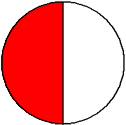The shaded part is 1/2 of the whole circle.This circle has been divided into 3 equal parts.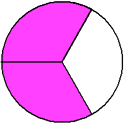The shaded part is 2/3 of the whole circle.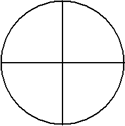This circle has been divided into 4 equal parts.The shaded part is 1/4 of the whole circle.

The numbers 1/2, 2/3 and 1/4 are called fractions, parts of the whole circle.

1/2 is pronounced as half or one over two, that’s one out of two.

2/3 as two third or two over three, two out of three.

1/4 as one quarter or one over four, one out of four.

The top number of a fraction is called a numerator and the bottom part is a denominator. Number of shaded parts is numerator while number of equal parts is denominator.

WORK – TO – DO

• Identify the name of the shaded parts of circles below:• Identify the name of the unshaded parts of circles below: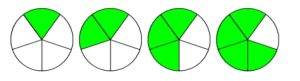LESSON TWO – EQUIVALENT FRACTIONS

Equivalent fractions are different fractions that name the same number.

Flash Back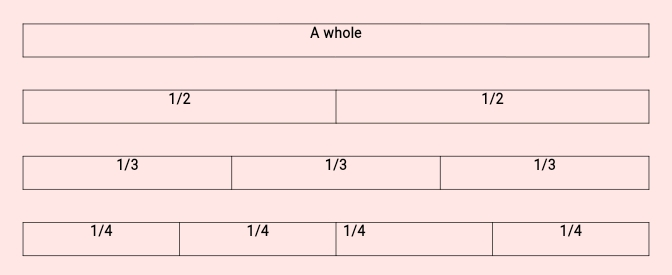Note – as the denominator get bigger, the fraction get smaller.

A.1/2

B.2/4

C.3/6

D.4/8

Each fraction in example 1 represents the same number. These are equivalent fractions.

That’s,

A = 1/2

B = (1 x 2)/(2 x 2) = 2/4

C = (1 x 3)/(2 x 3) = 1/6

D = (1 x 4)/(2 x 4) = 4/8

Reducing Fraction to its Lowest Term

When a fraction is divided by the same number until there is no common factor, we say that the fraction is in its lowest terms or in its simplest form.

For example,

Reduce:

1. 15/35
2. 20/30

15/35 = (3 x 5)/(7 x 5) = 3/7

20/30 = (2 x 10)/(3 x 10) = 2/3

WORK – TO – DO

Reducing the following fractions to their lowest term:

1. 8/24
2. 22/88
3. 64/72

LESSON THREE – ORDERING OF FRACTIONS (ASCENDING AND DESCENDING ORDERING)

• Flash Back

Put =, < or > in the brackets below:

1. 15  (   )  7
2. 13  (   )  19
3. 10  (   )  20/2

Arrange these numbers in ascending and descending order;

1. 23, 32, 12, 32, 21
2. 54, 46, 45, 64, 56

• ORDERING

Method 1 – Comparing fractions, if the denominators are the same.

Compare the fractions given below using the symbols <, > or =.

1. 1/8, 5/8
2. 7/10, 5/10
3. 1/5, 2/10, 3/10
• 1/8, 5/8

These fractions have like denominators, so we can compare the numerator, the larger fraction is the one with the greater numerator.

5 > 1and 1< 5

Therefore,

5/8 > 1/8 and 1/8 < 5/8

And also,

• 7/10 > 5/10  and 5/10 < 7/10
• 1/5 = 2/10 = 3/15

Comparing fractions if the denominators are not the same (find the LCM of the denominators)

Compare the fractions given below using the symbols < or >

2/3, 5/12, 1/4

• Denominators are 3, 12 and 4.

Multiple of 3 are 3, 6, 12, 15, 18…

Multiple of 12 are 12, 24, 36, 48…

Multiple of 4 are 4, 8, 12, 16, 20…

Common multiple is 12.

• Convert the fractions into equivalent fractions

2/3 = (2 x 4)/(3 x 4) = 8/12

5/12 = (5 x 1)/(12 x 1) = 5/12

1/4 = (1 x 3)/(4 x 3) = 3/12

Then, compare the numerators – 8, 5, 3.

8 > 5 > 3

Ascending order – 2/3 > 5/12 > 1/4

Descending order – 1/4 < 5/12 < 2/3

Method 2 – Fraction Bars

Put 1/4, 2/9, 1/7, 4/5Each divided bar presents the fractions – 1/4, 2/9, 1/7, 4/5.

You can now look at the shaded rectangles below and compare the bars.

1/4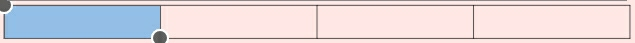2/9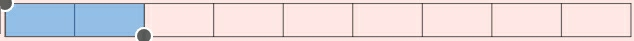1/74/5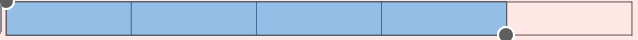The longest is the largest fraction, followed by the next until the last.

That’s,

1/7, 2/9, 1/4, 4/5 are in ascending order – smallest first.

4/5, 1/4, 2/9, 1/7 are in descending order – largest first.

WORK – TO – DO

Arrange in ascending and descending oder:

1. 1/5, 3/5, 2/5, 4/5
2. 2/7, 3/4, 5/6, 1/3

LESSON FOUR – ORDERING OF DECIMAL FRACTIONS

Method 4 – Convert the fractions into decimals.

Arrange 3/5, 1/100, 51/100, 25/100 in ascending order.

3/5 = 0.6

1/100 = 0.01

51/100 = 0.51

25/100 = 0.25

Now,

Arrange 0.6, 0.01, 0.51, 0.025 in ascending or descending order.

• Steps to ordering of decimals
1. Arrange the decimals in column.
2. Make the decimals the same length.
3. Ignore the decimal point.
4. Arrange in the order of size.
5. Replace with the corresponding decimal as illustrated below: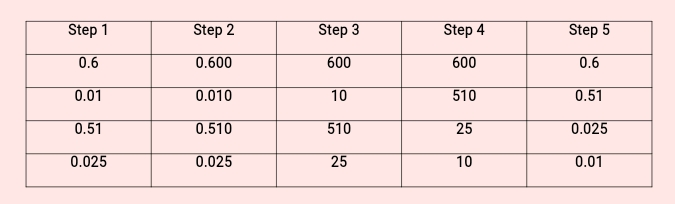Therefore,

Ascending order – 0.01, 0.025, 0.51, 0.6 or 1/100, 25/100, 51/100, 3/5.

WORK – TO – DO

1. 0.032, 0.2, 0.302, 0.3
2. 0.01, 0.11, 0.1, 0.101

PRESENTATION

• To deliver the lesson, the teacher adopts the following steps:
1. To introduce the lesson, the teacher revises the previous lesson. Based on this, he/she asks the pupils some questions;
2. Guides pupils to order fractions by first bringing the fractions to have the same denominator, consider the denominators and use other methods to arrange in ascending or descending order. ;
3. Pupil’s Activities – Order fractions by first bringing the fractions to have the same denominator, consider the denominators and use other methods to arrange in ascending or descending order.
4. Guides pupils to express decimals as fractions and vice versa;
5. Pupil’s Activities – Express decimals as fractions and vice versa.
6. Guides pupils to solve problems on quantitative aptitude e.g. Insert the appropriate symbol < or > in the diagram below;
7. Pupil’s Activities – Solve problems on quantitative reasoning involving fractions.

CONCLUSION

• To conclude the lesson for the week, the teacher revises the entire lesson and links it to the following week’s lesson.

LESSON EVALUATION

Pupils to:

1. order given fractions;
2. solve problems on quantitative aptitude involving fractions;
3. express given decimals as fractions and vice versa.

error: